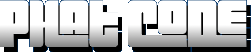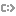If you hear an onion ring, answer it.

# Articles

## Graphics

 search for in All Assembly Audio Graphics Memory Collections Other Tutorials / References

## Alpha Blending Tutorial

 Author Toshi (Toshihiro Horie) Email horie@ocf.berkeley.edu Website http://www.ocf.berkeley.edu/~horie/project.html Released Jun 14 2002 PlatformLanguage QuickBASIC Summary Great tutorial from Toshi that covers alpha blending(aka "translucency") techniques. Example code in QuickBASIC.

# Alpha Blending Tutorial

### Introduction

What is alpha blending? It's a way of mixing the colors of two images together to form a final image. An example of naturally occuring alpha blending is a rainbow over a waterfall. If you think of the rainbow by itself as one image, and the background waterfall as another, then the final image can be formed by alpha blending the two together. Pixel artists and programmers sometimes call alpha blending "translucency"-- it is the same thing. In addition, when the blending factor changes dynamically over time, alpha blending is called "cross fade" or "dissolve." Here's an applet that does cross-fade: http://www.gaumentraum.de/sample/1.html

In this tutorial, we'll call the two input images as "source images," and the output image as the "blended image". The blending factor, or percentage of colors from the first source image used in the blended image is called the "alpha." The alpha used in algebra is in the range 0.0 to 1.0, instead of 0 to 100%, as in economics.

### The Algorithm

Alpha Blending can be accomplished in computer graphics by blending each pixel from the first source image with the corresponding pixel in the second source image. Here's the equation for doing the blending, first in English math, then in pseudocode.

Final pixel = alpha * (First image's source pixel) + (1.0-alpha) * (Second image's source pixel)

In a typical program, all the pixels in the above equation are in RGB888 format, or 8 bits for red, 8 bits for green, and 8 bits for blue color. If you layed out the bits from most significant on the left to least significant on the right, you'd get

bit 23                      bit 0
RRRRRRRRGGGGGGGGBBBBBBBB

You would define and alpha blend such a pixel in QuickBASIC like this:

DEFSNG A-Z

TYPE pixel
Red AS INTEGER
Green AS INTEGER
Blue AS INTEGER
END TYPE

DIM srcpixone AS pixel
DIM srcpixtwo AS pixel
DIM blendpix AS pixel

' source pixel one is purple
srcpixone.Red = 70
srcpixone.Green = 0
srcpixone.Blue = 80

' source pixel two is gray
srcpixtwo.Red = 120
srcpixtwo.Green = 120
srcpixtwo.Blue = 120

alpha = 0.3  ' 30 percent from srcpixone

' blendpix is the alpha blended pixel
blendpix.Red = srcpixone.Red * alpha + srcpixtwo.Red * (1.0 - alpha)
blendpix.Green = srcpixone.Green * alpha + srcpixtwo.Green * (1.0 - alpha)
blendpix.Blue = srcpixone.Blue * alpha + srcpixtwo.Blue * (1.0 - alpha)

' alpha blended pixel should be purplish gray

### Optimizations

Now, to make it faster, you can do several things. The first is to make a temporary variable for (1.0 - alpha). We'll call this invalpha.

alpha = 0.3  ' 30 percent from srcpixone
invalpha = 1.0 - alpha
' blendpix is the alpha blended pixel
blendpix.Red = srcpixone.Red * alpha + srcpixtwo.Red * invalpha
blendpix.Green = srcpixone.Green * alpha + srcpixtwo.Green * invalpha
blendpix.Blue = srcpixone.Blue * alpha + srcpixtwo.Blue * invalpha

The second optimization is to use fixed point math for alpha. For a description of fixed point math, read my rotation primer. We'll use 100 as the scale factor, so we can use percentages again!

DEFINT A-Z
DIM alpha, invalpha AS LONG
alpha = 30   '30 percent
invalpha = 100 - alpha
blendpix.Red = (srcpixone.Red * alpha + srcpixtwo.Red * invalpha) \ 100
blendpix.Green = (srcpixone.Green * alpha + srcpixtwo.Green * invalpha) \ 100
blendpix.Blue = (srcpixone.Blue * alpha + srcpixtwo.Blue * invalpha) \ 100

Now things should be pretty fast. But Blitz suggests a further optimization, which is to reduce the number of multiplies. The reason is because multiplies tend to take twice as long to execute than an addition or subtraction. He uses the mathematical fact that x*alpha + y*(1.0-alpha) can be shortened to alpha*(x-y) + y. This way, we don't need an extra variable for inverse alpha, either.

Proof:  x*a + y*(1-a)   =  x*a + y*(1) - y*a   =   x*a - y*a + y    =   (x-y)*a + y

DEFINT A-Z
DIM alpha AS LONG
alpha = 30   '30 percent
blendpix.Red = (srcpixone.Red - srcpixtwo.Red) * alpha + srcpixtwo.Red) \ 100
blendpix.Green = (srcpixone.Green - srcpixtwo.Green) * alpha + srcpixtwo.Green) \ 100
blendpix.Blue = (srcpixone.Blue - srcpixtwo.Blue) * alpha + srcpixtwo.Blue) \ 100

If you want it to go faster, you'd need to use table lookups, MMX, or bit shifts (to replace the slow divide operations) and other bitwise operations. If you want to learn more about these advanced methods, read http://www.stereopsis.com/xfade.html and http://www.stereopsis.com/doubleblend.html. Further, there are extremely fast ways to do 50% alpha blending (in one to five machine instructions per pixel). See http://space.virgilio.it/insignis@tin.it/tommesani/SSEPrimer.html and http://homepage1.nifty.com/herumi/adv/adv40.html for details.

### Displaying Alpha Blended Images

If you have a 24-bit SVGA library like Future.Library, http://www.qb45.com/, you wouldn't need much more than calling some PSET routine to code an alpha blender. However, if you are working in SCREEN 13, you have to deal with 24-bit to 8-bit paletted color conversion.

There are two strategies for color conversion. One is to try to get the best 256-color representation of the 24-bit image using the current palette. The other is to modify the 18-bit color palette and the pixels to get the best 256-color approximation possible.

For the first strategy (fixed palette), one algorithm is to precalculate the closest palette color for each point in the "quantized color cube." What the heck is a quantized color cube? Think of the red, green, and blue as separate axes of a 3 dimensional space. If we represented each of these primary colors with n shades, we would get n*n*n, or n^3 different colors. If we let n=7, we would get an RGBYYY representation, with Y=log2(7), having 343 distinct colors. Now, we try to find the closest color in our current palette to each of these 343 colors.

[What I mean by RGBYYY:]
By RGBYYY I mean, the display mode has Y bits for red, Y bits for green, and Y bits for the blue color component. For example, for a 24-bit display mode, Y would be 8; it can display 16777216 colors, and it has 2^8=256 shades of pure red, and 2^8 shades of pure green, and 2^8 shades of pure blue.There are several ways to measure "distance" between colors. The most familiar one should be the distance formula in three dimensions: dist = SQR((x1-x2)^2 + (y1-y2)^2 + (z1-z2)^2). But there are other ways of measuring distance. One way is called the "L1 norm," and you just do ABS(x1-x2) + ABS(y1-y2) + ABS(z1-z2). In the program, I used the L1 norm formula, but you can replace it with the distance formula if you wish.

'Uniform Colorcube Quantization routine
'...untested - use at your own risk....
DIM pal(0 TO 255) AS pixel

'read in the RGB888 representation of the current palette
QL = 7 ' number of quantization levels
FOR i=0 TO 255
OUT &amp;H3c7,i
pal(i).Red = INP(&amp;h3c9)*4
pal(i).Green = INP(&amp;h3c9)*4
pal(i).Blue = INP(&amp;h3c9)*4
NEXT

DIM bestcolor(QL-1,QL-1,QL-1)

'precalculation step

DIM quantcolor as pixel 'quantized color in color cube
FOR red=0 to QL-1
FOR grn=0 to QL-1
FOR blu=0 to QL-1
quantcolor.Red=red
quantcolor.Green=grn
quantcolor.Blue=blu
'initialize the minimum distance to a large number
mindiff = 255+255+255+1
bestindex = 0
FOR i=0 TO 255
' measure how different the current palette color is to the desired quantized color
dr = ABS(pal(i).Red-quantcolor.Red)
dg = ABS(pal(i).Red-quantcolor.Red)
db = ABS(pal(i).Red-quantcolor.Red)
d = (dr + dg + db)
IF d &lt; mindiff THEN
mindiff = d
bestindex=i
END IF
NEXT i
bestcolor(red,grn,blu) = bestindex
NEXT
NEXT
NEXT

'plot pixel step
DIM blendedimage(320,200) as pixel

FOR y=0 to 199
FOR x=0 to 319
rq = INT(blendedimage(x,y).Red * QL/256)
gq = INT(blendedimage(x,y).Green * QL/256)
bq = INT(blendedimage(x,y).Blue * QL/256)
'rq,gq, and bq should be in the range 0...6
PSET(x,y), bestcolor(rq,gq,bq)
NEXT x
NEXT y

There are other algorithms such as median cut and octree quantization http://www.cubic.org/~submissive/sourcerer/octree.htm that work better but run slower than this one.

The second strategy (modifying the palette) can be combined with the uniform color cube quantization, by selecting palette entries with colors close to the center of each shade of color within the quantized color cube.